## Thursday, February 17, 2011

### Spatial indexing with Quadtrees and Hilbert Curves

Last Thursday night at Oredev, after the sessions, was "Birds of a Feather" - a sort of mini-unconference. Anyone could write up a topic on the whiteboard; interested individuals added their names, and each group got allocated a room to chat about the topic. I joined the "Spatial Indexing" group, and we spent a fascinating hour and a half talking about spatial indexing methods, reminding me of several interesting algorithms and techniques.
Spatial indexing is increasingly important as more and more data and applications are geospatially-enabled. Efficiently querying geospatial data, however, is a considerable challenge: because the data is two-dimensional (or sometimes, more), you can't use standard indexing techniques to query on position. Spatial indexes solve this through a variety of techniques. In this post, we'll cover several - quadtrees, geohashes (not to be confused with geohashing), and space-filling curves - and reveal how they're all interrelated.

Quadtrees are a very straightforward spatial indexing technique. In a Quadtree, each node represents a bounding box covering some part of the space being indexed, with the root node covering the entire area. Each node is either a leaf node - in which case it contains one or more indexed points, and no children, or it is an internal node, in which case it has exactly four children, one for each quadrant obtained by dividing the area covered in half along both axes - hence the name.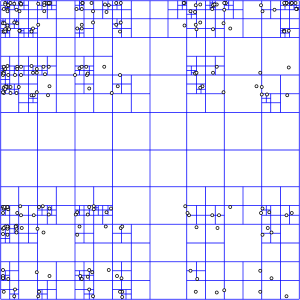A representation of how a quadtree divides an indexed area. Source: Wikipedia
Inserting data into a quadtree is simple: Starting at the root, determine which quadrant your point occupies. Recurse to that node and repeat, until you find a leaf node. Then, add your point to that node's list of points. If the list exceeds some pre-determined maximum number of elements, split the node, and move the points into the correct subnodes.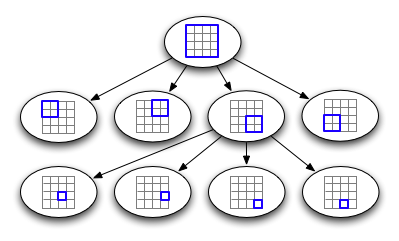A representation of how a quadtree is structured internally.
To query a quadtree, starting at the root, examine each child node, and check if it intersects the area being queried for. If it does, recurse into that child node. Whenever you encounter a leaf node, examine each entry to see if it intersects with the query area, and return it if it does.
Note that a quadtree is very regular - it is, in fact, a trie, since the values of the tree nodes do not depend on the data being inserted. A consequence of this is that we can uniquely number our nodes in a straightforward manner: Simply number each quadrant in binary (00 for the top left, 10 for the top right, and so forth), and the number for a node is the concatenation of the quadrant numbers for each of its ancestors, starting at the root. Using this system, the bottom right node in the sample image would be numbered 11 01.
If we define a maximum depth for our tree, then, we can calculate a point's node number without reference to the tree - simply normalize the node's coordinates to an appropriate integer range (for example, 32 bits each), and then interleave the bits from the x and y coordinates -each pair of bits specifies a quadrant in the hypothetical quadtree.

### Geohashes

This system might seem familiar: it's a geohash! At this point, you can actually throw out the quadtree itself - the node number, or geohash, contains all the information we need about its location in the tree. Each leaf node in a full-height tree is a complete geohash, and each internal node is represented by the range from its smallest leaf node to its largest one. Thus, you can efficiently locate all the points under any internal node by indexing on the geohash by performing a query for everything within the numeric range covered by the desired node.
Querying once we've thrown away the tree itself becomes a little more complex. Instead of refining our search set recursively, we need to construct a search set ahead of time. First, find the smallest prefix (or quadtree node) that completely covers the query area. In the worst case, this may be substantially larger than the actual query area - for example, a small shape in the center of the indexed area that intersects all four quadrants would require selecting the root node for this step.
The aim, now, is to construct a set of prefixes that completely covers the query region, while including as little area outside the region as possible. If we had no other constraints, we could simply select the set of leaf nodes that intersect the query area - but that would result in a lot of queries. Another constraint, then, is that we want to minimise the number of distinct ranges we have to query for.
One approach to doing this is to start by setting a maximum number of ranges we're willing to have. Construct a set of ranges, initially populated with the prefix we identified earlier. Pick the node in the set that can be subdivided without exceeding the maximum range count and will remove the most unwanted area from the query region. Repeat this until there are no ranges in the set that can be further subdivided. Finally, examine the resulting set, and join any adjacent ranges, if possible. The diagram below demonstrates how this works for a query on a circular area with a limit of 5 query ranges.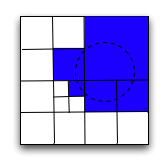How a query for a region is broken into a series of geohash prefixes/ranges.
This approach works well, and it allows us to avoid the need to do recursive lookups - the set of range lookups we do execute can all be done in parallel. Since each lookup can be expected to require a disk seek, parallelizing our queries allows us to substantially cut down the time required to return the results.
Still, we can do better. You may notice that all the areas we need to query in the above diagram are adjacent, yet we can only merge two of them (the two in the bottom right of the selected area) into a single range query, requiring us to do 4 separate queries. This is due in part to the order that our geohashing approach 'visits' subregions, working left to right, then top to bottom in each quad. The discontinuity as we go from top right to bottom left quad results in us having to split up some ranges that we could otherwise make contiguous. If we were to visit regions in a different order, perhaps we could minimise or eliminate these discontinuities, resulting in more areas that can be treated as adjacent and fetched with a single query. With an improvement in efficiency like that, we could do fewer queries for the same area covered, or conversely, the same number of queries, but including less extraneous area.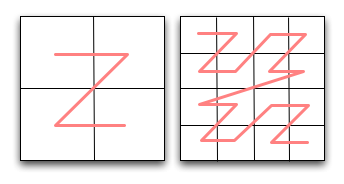Illustrates the order in which the geohashing approach 'visits' each quad.

### Hilbert Curves

Suppose instead, we visit regions in a 'U' shape. Within each quad, of course, we also visit subquads in the same 'U' shape, but aligned so as to match up with neighbouring quads. If we organise the orientation of these 'U's correctly, we can completely eliminate any discontinuities, and visit the entire area at whatever resolution we choose continuously, fully exploring each region before moving on to the next. Not only does this eliminate discontinuities, but it also improves the overall locality. The pattern we get if we do this may look familiar - it's a Hilbert Curve.
Hilbert Curves are part of a class of one-dimensional fractals known as space-filling curves, so named because they are one dimensional lines that nevertheless fill all available space in a fixed area. They're fairly well known, in part thanks to XKCD's use of them for a map of the internet. As you can see, they're also of use for spatial indexing, since they exhibit exactly the locality and continuity required. For example, if we take another look at the example we used for finding the set of queries required to encompass a circle above, we find that we can reduce the number of queries by one: the small region in the lower left is now contiguous with the region to its right, and whilst the two regions at the bottom are no longer contiguous with each other, the rightmost one is now contiguous with the large area in the upper right.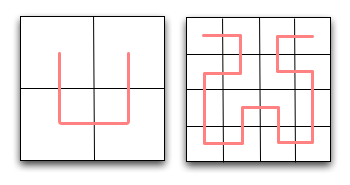Illustrates the order in which a hilbert curve 'visits' each quad.
One thing that our elegant new system is lacking, so far, is a way of converting between a pair of (x,y) coordinates and the corresponding position in the hilbert curve. With geohashing it was easy and obvious - just interleave the x and y coordinates - but there's no obvious way to modify that for a hilbert curve. Searching the internet, you're likely to come across many descriptions of how hilbert curves are drawn, but few if any descriptions of how to find the position of an arbitrary point. To figure this out, we need to take a closer look at how the hilbert cure can be recursively constructed.
The first thing to observe is that although most references to hilbert curves focus on how to draw the curve, this is a distraction from the essential property of the curve, and its importance to us: It's an ordering for points on a plane. If we express a hilbert curve in terms of this ordering, drawing the curve itself becomes trivial - simply a matter of connecting the dots. Forget about how to connect adjacent sub-curves, and instead focus on how we can recursively enumerate the points.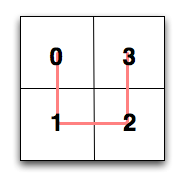Hilbert curves are all about ordering a set of points on a 2d plane
At the root level, enumerating the points is simple: Pick a direction and a start point, and proceed around the four quadrants, numbering them 0 to 3. The difficulty is introduced when we want to determine the order we visit the sub-quadrants in while maintaining the overall adjacency property. Examination reveals that each of the sub-quadrants' curves is a simple transformation of the original curve: there are only four possible transformations. Naturally, this applies recursively to sub-sub quadrants, and so forth. The curve we use for a given quadrant is determined by the curve we used for the square it's in, and the quadrant's position. With a little work, we can construct a table that encapsulates this: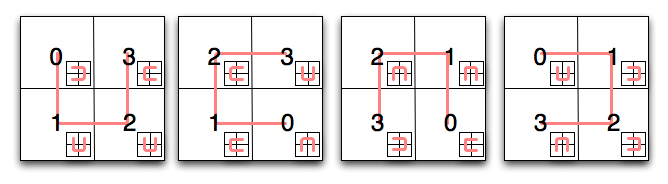Suppose we want to use this table to determine the position of a point on a third-level hilbert curve. For the sake of this example, assume our point has coordinates (5,2) Starting with the first square on the diagram, find the quadrant your point is in - in this case, it's the upper right quadrant. The first part of our hilbert curve position, then, is 3 (11 in binary). Next, we consult the square shown in the inset of square 3 - in this case, it's the second square. Repeat the process: which sub-quadrant does our point fall into? Here, it's the lower left one, meaning the next part of our position is 1, and the square we should consult next is the second one again. Repeating the process one final time, we find our point falls in the upper right sub-sub-quadrant, our final coordinate is 3 (11 in binary). Stringing them together, we now know the position of our point on the curve is 110111 binary, or 55.
Let's be a little more methodical, and write methods to convert between x,y coordinates and hilbert curve positions. First, we need to express our diagram above in terms a computer can understand:
`hilbert_map = { 'a': {(0, 0): (0, 'd'), (0, 1): (1, 'a'), (1, 0): (3, 'b'), (1, 1): (2, 'a')}, 'b': {(0, 0): (2, 'b'), (0, 1): (1, 'b'), (1, 0): (3, 'a'), (1, 1): (0, 'c')}, 'c': {(0, 0): (2, 'c'), (0, 1): (3, 'd'), (1, 0): (1, 'c'), (1, 1): (0, 'b')}, 'd': {(0, 0): (0, 'a'), (0, 1): (3, 'c'), (1, 0): (1, 'd'), (1, 1): (2, 'd')}, }` In the snippet above, each element of 'hilbert_map' corresponds to one of the four squares in the diagram above. To make things easier to follow, I've identified each one with a letter - 'a' is the first square, 'b' the second, and so forth. The value for each square is a dict, mapping x and y coordinates for the (sub-)quadrant to the position along the line (the first part of the value tuple) and the square to use next (the second part of the value tuple). Here's how we can use this to translate x and y coordinates into a hilbert curve position:
`def point_to_hilbert(x, y, order=16): current_square = 'a' position = 0 for i in range(order - 1, -1, -1): position <<= 2 quad_x = 1 if x & (1 << i) else 0 quad_y = 1 if y & (1 << i) else 0 quad_position, current_square = hilbert_map[current_square][(quad_x, quad_y)] position |= quad_position return position` The input to this function is the integer x and y coordinates, and the order of the curve. An order 1 curve fills a 2x2 grid, an order 2 curve fills a 4x4 grid, and so forth. Our x and y coordinates, then, should be normalized to a range of 0 to 2order-1. The function works by stepping over each bit of the x and y coordinates, starting with the most significant. For each, it determines which (sub-)quadrant the coordinate lies in, by testing the corresponding bit, then fetches the position along the line and the next square to use from the table we defined earlier. The curve position is set as the least significant 2 bits on the position variable, and at the beginning of the next loop, it's left-shifted to make room for the next set of coordinates.
Let's check that we've written the function correctly by running our example from above through it:
```>>> point_to_hilbert(5,2,3) 55``` Presto! For a further test, we can use the function to generate a complete list of ordered points for a hilbert curve, then use a spreadsheet to graph them and see if we get a hilbert curve. Enter the following expression into an interactive Python interpreter:
```>>> points = [(x, y) for x in range(8) for y in range(8)] >>> sorted_points = sorted(points, key=lambda k: point_to_hilbert(k, k, 3)) >>> print '\n'.join('%s,%s' % x for x in sorted_points)                         ``` Take the resulting text, paste it into a file called 'hilbert.csv', open it in your favorite spreadsheet, and instruct it to generate a scatter plot. The result is, of course, a nicely plotted hilbert curve!
The inverse of point_to_hilbert is a straightforward reversal of the hilbert_map; implementing it is left as an exercise for the reader.

### Conclusion

There you have it - spatial indexing, from quadtrees to geohashes to hilbert curves. One final observation: If you express the ordered sequence of x,y coordinates required to draw a hilbert curve in binary, do you notice anything interesting about the ordering? Does it remind you of anything?
Just to wrap up, a caveat: All of the indexing methods I've described today are only well-suited to indexing points. If you want to index lines, polylines, or polygons, you're probably out of luck with these methods - and so far as I'm aware, the only known algorithm for effectively indexing shapes is the R-tree, an entirely different and more complex beast.

### Anagram Trees : Part 2

One nice thing about working at Google is that you are surrounded by very smart people. I told one of my coworkers about the anagram tree idea, and he immediately pointed out that reordering the alphabet so that the least frequently used letters come first would reduce the branching factor early in the tree, which has the effect of reducing the overall size of the tree substantially. While this seems obvious in retrospect, it's kind of unintuitive - usually we try to _increase_ the branching factor of n-ary trees to make them shallower and require fewer operations, rather than trying to reduce it.

Trying it out with an ordering determined by looking at the branching factor for each letter produces results that bear this out: Memory is reduced by about a third, and the number of internal nodes is reduced to 858,858 from 1,874,748, a reduction of more than 50! Though I haven't benchmarked it, difficult lookups are substantially faster, too.

The next logical development to try is to re-evaluate the order of the alphabet on a branch-by-branch basis. While I doubt this will have a substantial impact, it seems worth a try, so I'll give it a go and update with results.

Edit: Re-evaluating the symbol to choose on a branch-by-branch basis had a bigger impact than I anticipated: The tree created with my sample dictionary now has a mere 661,659 internal nodes. Here's the procedure for creating a tree using this method:

Assuming you have:
• A dictionary
• A set of symbols that have not yet been used (initially set to the alphabet)
1. If the symbol set is empty, this is a leaf node - store the dictionary in the node and return.
2. Find the symbol from the set that, if used, will result in the smallest number of branches (that is, the symbol that has the least variation in number of occurrences).
3. Mark the current node with the chosen symbol
4. Partition the dictionary into sub-dictionaries based on how many occurrences of the chosen symbol they have
5. For each sub-dictionary, recurse with the sub-dictionary and the set less the symbol you selected.
Implemented in Python, this is actually substantially larger in memory and on disk than the previous approach, likely due to overhead with using classes instead of tuples as the nodes. In statically-typed languages, however, the overhead should be substantially outweighed by the benefit of the reduction in node count.

Note that the result of this alternate method is that while the letter to branch on is different for every node, following nodes from any leaf to the root of the tree always results in a valid permutation of the alphabet used.

Edit 2: The code for a Python implementation incorporating these ideas can be found here

### Anagram Trees

When it comes to finding anagrams of words, a frequent approach is to use an anagram dictionary - simply put, sort the letters in your word to provide a unique key that all anagrams of a word have in common. Another approach is to generate a letter-frequency histogram for each letter in your word. (Both these approaches are more or less equivalent, in fact.) These approaches make the problem of finding exact single-word anagrams for strings very efficient - O(1) if you use a hashtable.

However, the problem of finding subset anagrams - a word that contains a subset of the letters in a string - is still rather inefficient, requiring either a brute force O(n) search through the dictionary, or looking up every substring of the sorted input string, which is O(2^l) with the number of letters in the input string. Finding subset anagrams is significantly more interesting, too, as it has applications in finding multi-word anagrams, as well as being applicable to problem domains such as scrabble.

However, with a little more effort, and the above observation that we can generate a histogram that uniquely represents a given set of letters, we can generate a tree structure that makes looking up subset anagrams much more efficient. To build the tree, we follow this simple procedure:

Assume we have the following information:
• A lexicon or dictionary of words to populate the tree with
• An alphabet for words in the lexicon
• The tree we are building
• A current node
For each term in the lexicon:
1. Generate a letter-frequency histogram for the term.
2. Set the current node to the root of the tree.
3. For each symbol in the alphabet:
1. Get the frequency of the current symbol in the current term. Call it f
2. Set the current node to the fth child node of the current node, creating it if it doesn't exist
4. Append the current term to the list of words on the current (leaf) node

The result of following this simple procedure is a fixed-height tree, 27 nodes deep, with all the words in the leaf nodes, and each internal tier of the tree corresponding to a symbol from the alphabet. Here's an (abbreviated) example:Once the tree is built, we find subset anagrams for an input string as follows:

Assume we have the following information:
• The tree we built using the above procedure.
• The alphabet we used above.
• A frontier set, initially empty.
1. Initialize the frontier set to contain the root of the tree.
2. Generate a letter-frequency histogram for the input string.
3. For each symbol in the alphabet:
1. Get the frequency of the current symbol in the input string. Call it f.
2. For each node in the current frontier set, add the subnodes numbered 0 through f to the new frontier set.
4. The frontier set now consists of leaf nodes, containing all the subset anagrams of the input string.

Runtime analysis of this algorithm is rather difficult, for me, at least. Intuitively and in practice, it's a lot faster than either of the brute-force approaches, but quantifying that in big-O notation is something that's escaped me. As an upper bound, it cannot be less efficient than O(n) - only a constant factor worse than the brute-force approach. As a lower bound, a lookup in which the frontier set always has one node, lookup time is proportional to the length of the alphabet, or O(1). The average case depends on how large a subset of the dictionary the input string selects. Quantifying by the size of the output, approximately O(m) operations are required. If anyone knows how to determine more solid bounds for runtime, please do let me know in the comments.

One disadvantage of this approach is that there is substantial memory overhead. Using my Python implementation of the algorithm, and importing /usr/share/dict/words, which is approximately 2MB on this machine results in over 300MB of memory consumed. Using the Pickle module to serialize to disk, the output file is over 30MB, and compresses with gzip down to about 7MB. I suspect part of the large memory overhead is due to the minimum size of Python's dictionaries; I will modify the implementation to use lists and update this post if I can make it more efficient.

Here's a few stats on the tree generated that may be of interest:
Total words: 234,936
Leaf nodes: 215,366
Internal nodes: 1,874,748

From this we can see that the average cardinality of internal nodes is very low - not much more than 1. A breakdown of the number of nodes in each tier helps clarify this:
TierNumber of nodes
0 1
1 7
2 25
3 85
4 203
5 707
6 1145
7 1886
8 3479
9 8156
10 8853
11 10835
12 19632
13 28470
14 47635
15 73424
16 92618
17 94770
18 125018
19 156406
20 182305
21 195484
22 200031
23 203923
24 205649
25 214001

The cardinality of nodes towards the top of the tree is fairly high, but the tree quickly flattens out, and the last four tiers of the tree account for almost half of the total nodes. This suggests one possible space optimisation: Removing the last few tiers of the tree and concatenating their leaf nodes together. When performing lookups, check the selected nodes to ensure they are actually subset anagrams of the input string.

* It's possible I'm simply rediscovering something that's well known in the computer science community, or perhaps mentioned in a computer science paper 30 years ago. Significant searching hasn't turned up anyone using an algorithm like this, or anything else more efficient than the brute-force approaches outlined.

Edit: The source to my initial implementation is here.

Edit: Converting my Python implementation to use lists reduced memory consumption by roughly half. I'll post figures for the pickled tree and the source code when I have the opportunity.

Edit: More updates can be found here.

### Secure permutations with block ciphers

It's been too long since I blogged about anything much, and way too long since I posted the first Damn Cool Algorithms post, which I promised would be a series. So here's part 2.

To start, I'm assuming you know what a permutation is - basically a shuffling of a sequence of items in a particular order. A permutation of the range 1-10, for example, is {5,2,1,6,8,4,3,9,7,10}. A secure permutation is one in which an attacker, given any subset of the permutation, cannot determine the order of any other elements. A simple example of this would be to take a cryptographically secure pseudo-random number generator, seed it with a secret key, and use it to shuffle your sequence.

What if you want to generate a really, really big permutation - one so big precomputing and storing it isn't practical or desirable? Furthermore, what if you want it to be a secure permutation? There's a really neat trick we can pull with block ciphers that allows us to generate a secure permutation over any range of numbers without first having to precompute it.

A block cipher, for anyone that isn't familiar with them, is a common cryptographic primitive. It takes blocks of ciphertext of some fixed lengths - 64 or 128 bits is common - and encrypts it. Given the same key and the same block of plaintext, it will always generate the same block of ciphertext. Messages larger than a single block are encrypted using one of a number of modes of operation, allowing messages much larger than a single block to be encrypted and decrypted securely. When using a block cipher for encryption, choice of the mode of operation is critical. For the purposes of generating a secure permutation, however, we're only going to be encrypting a single block at a time, so we don't have to worry about modes of operation.

If you look at how a block cipher operates - taking any block of a given length (think of blocks as very large numbers here) and converting it uniquely to another block, such that it can be converted back again, a block cipher is already a secure permutation. If we progressively encrypt larger numbers (1, 2, 3, and so on), we get out a random seeming sequence of output numbers that is guaranteed not to repeat as long as we don't repeat our input. It's easy to prove this to yourself: If it repeated, then you would have two input numbers with a single output number, and it would thus be impossible to decrypt uniquely. So the same properties that a block cipher requires are the properties that make it useful to us.

All very well, you say, but what if I want a permutation over a range that isn't a power of two? This is where the clever trick comes in. Take a block cipher that's got a block length slightly larger than you want. Use it as described above, encrypting progressively higher numbers in a sequence to generate elements in the permutation. Whenever the output of the encryption is outside the range you want for your permutation, just encrypt it again. Repeat until you get a number within the range you want. Again, we're guaranteed uniqueness by the block cipher, and we're also guaranteed (by exhaustion) that we will eventually get a number within the desired range.

Obviously, there are some factors that need to be taken into consideration before pursuing this route. You want to select a block cipher that is not much larger than the range you wish to generate a permutation over - preferably the next power of two. The ratio of the cipher's range to the permutation's range defines the average amount of work you will have to perform, so if the cipher has a range four times that of your permutation, you'll need to do an average of four encryptions for each value. Since most block ciphers are 64, 128, or more bits, this can be problematic. For this purpose, I've found the TEA cipher to be particularly adaptable. It is easy to create variants that are 32, 64, 128 or more bits long, and from there, the bitshifts in the main loop are easily adjusted to produce a cipher with a length that's any power of 4, without needing to shorten the key to the point where it's easily brute-forced.

It's also worth noting that although this technique is aimed at generating very large secure permutations, it is equally useful for a permutation that doesn't need to be secure or secret - your secret key simply becomes your random seed for the permutation. There are many situations in which this can be useful - what you essentially have is a mapping function from index to permutation value, so you can calculate the value of any subset of the permutation that you wish.

Finally, bear in mind that due to the factorial explosion of the number of possible permutations, the keyspace of your cipher is almost certainly going to be much smaller than the number of possible permutations. For most purposes this probably does not matter, since the number of possible permutations is too large to enumerate anyway, but if your key is sufficiently short, it allows the possibility of an attacker doing an exhaustive search of your keyspace to find the permutation that generates the subsequence of the permutation he has access to.

Update: Yossi Oren points to this excellent paper in the comments. It covers exactly what I describe here (only much more comprehensively, of course).

### BK-Treesal

I am making my own vocab software. I provided words, meanings, synonyms, search. I feel the project is partially over. So now I thought, why not provide google-like "Did you mean" option in my search.
So it all starts with this, I came closer to algorithms again. :)

BK-Trees
BK-Trees, or Burkhard-Keller Trees are a tree-based data structure engineered for quickly finding near-matches to a string, for example, as used by a spelling checker, or when doing a 'fuzzy' search for a term. The aim is to return, for example, "seek" and "peek" if I search for "aeek". What makes BK-Trees so cool is that they take a problem which has no obvious solution besides brute-force search, and present a simple and elegant method for speeding up searches substantially.

BK-Trees were first proposed by Burkhard and Keller in 1973, in their paper "Some approaches to best match file searching". The only copy of this online seems to be in the ACM archive, which is subscription only. Further details, however, are provided in the excellent paper "Fast Approximate String Matching in a Dictionary".

Before we can define BK-Trees, we need to define a couple of preliminaries. In order to index and search our dictionary, we need a way to compare strings. The canonical method for this is the Levenshtein Distance, which takes two strings, and returns a number representing the minimum number of insertions, deletions and replacements required to translate one string into the other. Other string functions are also acceptable (for example, one incorportating the concept of transpositions as an atomic operation could be used), as long as they meet the criteria defined below.

Now we can make a particularly useful observation about the Levenshtein Distance: It forms a Metric Space. Put simply, a metric space is any relationship that adheres to three basic criteria:
• d(x,y) = 0 <-> x = y (If the distance between x and y is 0, then x = y)
• d(x,y) = d(y,x) (The distance from x to y is the same as the distance from y to x)
• d(x,y) + d(y,z) >= d(x,z)

The last of these criteria is called the Triangle Inequality. The Triangle Inequality states that the path from x to z must be no longer than any path that goes through another intermediate point (the path from x to y to z). Look at a triangle, for example: it's not possible to draw a triangle such that it's quicker to get from one point to another by going along two sides than it is by going along the other side.

These three criteria, basic as they are, are all that's required for something such as the Levenshtein Distance to qualify as a Metric Space. Note that this is far more general than, for example, a Euclidian Space - a Euclidian Space is metric, but many Metric Spaces (such as the Levenshtein Distance) are not Euclidian. Now that we know that the Levenshtein Distance (and other similar string distance functions) embodies a Metric Space, we come to the key observation of Burkhard and Keller.

Assume for a moment we have two parameters, query, the string we are using in our search, and n the maximum distance a string can be from query and still be returned. Say we take an arbitary string, test and compare it to query. Call the resultant distance d. Because we know the triangle inequality holds, all our results must have at most distance d+n and at least distance d-n from test.

From here, the construction of a BK-Tree is simple: Each node has a arbitrary number of children, and each edge has a number corresponding to a Levenshtein distance. All the subnodes on the edge numbered n have a Levenshtein distance of exactly n to the parent node. So, for example, if we have a tree with parent node "book" and two child nodes "rook" and "nooks", the edge from "book" to "rook" is numbered 1, and the edge from "book" to "nooks" is numbered 2.

To build the tree from a dictionary, take an arbitrary word and make it the root of your tree. Whenever you want to insert a word, take the Levenshtein distance between your word and the root of the tree, and find the edge with number d(newword,root). Recurse, comparing your query with the child node on that edge, and so on, until there is no child node, at which point you create a new child node and store your new word there. For example, to insert "boon" into the example tree above, we would examine the root, find that d("book", "boon") = 1, and so examine the child on the edge numbered 1, which is the word "rook". We would then calculate the distance d("rook", "boon"), which is 2, and so insert the new word under "rook", with an edge numbered 2.

To query the tree, take the Levenshtein distance from your term to the root, and recursively query every child node numbered between d-n and d+n (inclusive). If the node you are examining is within d of your search term, return it and continue your query.

The tree is N-ary and irregular (but generally well-balanced). Tests show that searching with a distance of 1 queries no more than 5-8% of the tree, and searching with two errors queries no more than 17-25% of the tree - a substantial improvement over checking every node! Note that exact searching can also be performed fairly efficiently by simply setting n to 0.
Thats it for now.

## Sunday, February 6, 2011

### Why is manhole cover circular?

Reasons for the shape include:
• A round manhole cover cannot fall through its circular opening, whereas a square manhole cover may fall in if it were inserted diagonally in the hole. (AReuleaux triangle or other curve of constant width would also serve this purpose, but round covers are much easier to manufacture. The existence of a "lip" holding up the lid means that the underlying hole is smaller than the cover, so that other shapes might suffice.)
• Round tubes are the strongest and most material-efficient shape against the compression of the earth around them, and so it is natural that the cover of a round tube assume a circular shape.
• Similarly, it is easier to dig a circular hole and thus the cover is also circular.
• The bearing surfaces of manhole frames and covers are machined to assure flatness and prevent them from becoming dislodged by traffic. Round castings are much easier to machine using a lathe.
• Circular covers do not need to be rotated to align them when covering a circular manhole.
• Human beings have a roughly circular cross-section.
• A round manhole cover can be more easily moved by being rolled.
• If a cover had corners and were bent that would create a protruding point that could puncture tires.
• Most manhole covers are made by a few large companies. A different shape would have to be custom made.